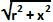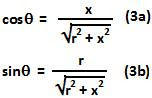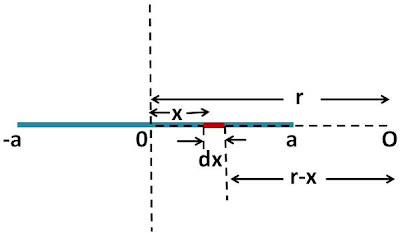## Monday, February 1, 2010

### Irodov Problem 3.13

a)Consider an infinitesimally small section of the rod of length dx that is at a distance x from the center of the rod. The charge contained in this infintesimally small section of the rod will beThe distance of this infinitesimally small section from point O is given by. The electric field generated by this infinitesimally small section of the rod is thus given by,From elemetary geometry we know that,From (1),(2), (3a) and (3b) we have,b)Consider an infinitesimally small section of the rod of length dx that is at a distance x from the center of the rod. The charge contained in this infinitesimally small section of the rod is given by (1). The distance of this section from O is given by r-x. Hence, we have,